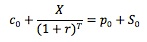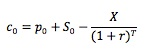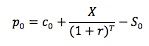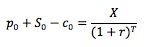### Why should I choose AnalystNotes?

AnalystNotes specializes in helping candidates pass. Period.

### Subject 1. Fiduciary calls and protective puts

First we consider an option strategy referred to as a fiduciary call which consists of a European call and a risk-free bond that matures on the option expiration day and has a face value (X) equal to the exercise price of the call.

• If the price of the underlying is below X at expiration, the call expires worthless and the bond is worth X.
• If the price of the underlying is above X at expiration, the call expires and is worth ST (the underlying price) - X.

So at expiration, the fiduciary call will end us with X or ST, whichever is greater. This combination allows protection against downside losses and is thus faithful to the notion of preserving capital.

Then we consider an option strategy known as a protective put, which consists of a European put and the underlying asset.

• If the price of the underlying is below X at expiration, the put expires and is worth X - ST, and the underlying is worth ST.
• If the price of the underlying is above X at expiration, the put expires with no value and the underlying is worth ST.

So at expiration, the protective put is worth X or ST, whichever is greater.

Thus, the fiduciary call and protective put end up with the same value. They are therefore identical combinations. To avoid arbitrage their values today must be the same.This equation is called put-call parity. It does not say the puts and calls are equivalent, but it does show an equivalence (parity) of a call/bond portfolio and a put/underlying portfolio. Note that the put and call must have the same underlying, exercise price and expiration date.

By re-arranging the above equation:Because the right side of this equation is equivalent to a call, it is often referred to as a synthetic call. It consists of a long put, a long position in the underlying, and a short position in the risk-free bond.

There are numerous other combinations that can be constructed. For example, we can isolate the put as:The right side is a synthetic put, which consists of a long call, a short position in the underlying, and a long position in the risk-free bond.

Another example is synthetic bond:Synthetic positions enable us to price options, because they produce the same results as options and have known prices. Consider the following example: A European call with an exercise price of \$30 expires in 90 days. A European put with the same exercise price, expiration date and underlying is selling for \$6. The underlying is selling for \$40, and the risk-free rate is 10%. Based on the information, we can compute the value of the synthetic call: c0 = p0 + S0 - X/(1 + r)T = 6 + 40 - 30/(1 + 10%)90/365 = \$16.7 (Note the time to expiration T = 90/365 = 0.2466.). Since the synthetic call and the actual call have the same payoff, they must have the same price as well. Therefore, the price of the call should be \$16.70.

Synthetic positions also tell how to exploit mis-pricing of options relative to their underlying assets. Continue with the above example. If the market price of the call is \$10, the call is then under-priced. We can make a risk-free profit of \$6.70 by selling the synthetic call for \$16.7.

We can not only synthesize a call or a put, but also synthesize the underlying or the bond.

#### Practice Question 1

Suppose an investor buys one share of stock and a put option on the stock. What will be the value of her investment on the final exercise date if the stock price is below the exercise price?

A. The value of two shares of stock
B. The value of one share of stock minus the exercise price
C. The exercise price.

#### Practice Question 2

Suppose an investor buys one share of stock and a put option on the stock and simultaneously sells a call option on the stock with the same exercise price. What will be the value of his investment on the final exercise date?

A. Above the exercise price if the stock price rises and below the exercise price if it falls.
B. Equal to the exercise price regardless of the stock price.
C. Equal to zero regardless of the stock price.

#### Practice Question 3

Dolores has studied payoff tables to determine the possible payoffs from buying a call option. This exercise will help her determine:

A. whether to purchase an American or European option.
B. the cash outflow from the possible outcomes.
C. the underlying stocks volatility.
D. the price to pay for the option.

Payoff tables are studied to determine the price to pay for the option.

#### Practice Question 4

An investor buys shares of ABC Corp. at \$30 and immediately writes a call on them. If the call carries a premium of \$2.5 and its exercise price is \$30, at what price of ABC shares will this investor break-even?

A. \$27.50.
B. \$32.50.
C. Call options cannot have a negative value, therefore, there is no break-even point.

A call is at the money when its exercise price is equal to the current price of the asset. In this case, both the exercise price and the asset price is \$30.

A covered call writer refers to an investor who owns the underlying asset and simultaneously sells (or writes) a call option. If the investor is writing the call, he will receive a premium of \$2.50.

Should the asset price close above \$30 before expiration, the writer of the call will have to deliver his stock. He loses the stock, but walks away with the premium he made.

On the other hand, if the stock price closes below \$30, the writer will not be called upon to deliver the stock. However, the dropping stock price implies that he is losing value on the stock. If the stock price drops to \$27.50, he has lost \$2.50 on his stock; but since he made \$2.50 selling the call in the beginning, he breaks even. Should the stock price drop below \$27.50, his losses will offset any money he made selling the call. Hence, \$27.50 is the break-even point.

#### Practice Question 5

Your research department has just made a sound presentation arguing that the equity markets are due for a sever correction in the short term. Which of the following strategies would be most suitable to safeguard the portfolio that you manage?

A. Long futures contacts on an equity index.
B. Buy put options on an equity index.

If you expect stock prices to decline, then you'll want to enter into an agreement that will allow you to sell shares at a pre-determined price. This way, you are effectively locking in the future value of the shares. There are only two strategies that will enable you to carry out this transaction. First, buying a put will give you the "right" to deliver these shares at a pre-determined price. And second, selling stock index futures will "obligate" you to deliver these shares at a pre-determined price.

#### Practice Question 6

Which of the following strategies would you advise against the most if you were expecting stock prices to appreciate significantly?

A. Writing a put while owning the underlying asset.
B. Writing a put without actually owning the underlying asset.
C. Writing calls without actually owning the underlying asset.

If stock prices are expected to increase, it would be very risky to sell securities that appreciate in value due to a market appreciation. Writing a call option would be exactly that. Uncovered call writing simply refers to the practice of selling a call when the underlying stock is not owned by the investor.

#### Practice Question 7

Which of the following statements is (are) true with respect to insuring a portfolio by way of protective strategy?

I. The strategy requires the sale of put options while owning the underlying asset.
II. If the price of the underlying asset increases dramatically, then an insured portfolio will perform much better than an uninsured portfolio.
III. The cost of portfolio insurance will rise as the volatility of the underlying asset increases.
IV. The upside potential for an insured portfolio is unlimited.

A. I and IV
B. III only
C. III and IV